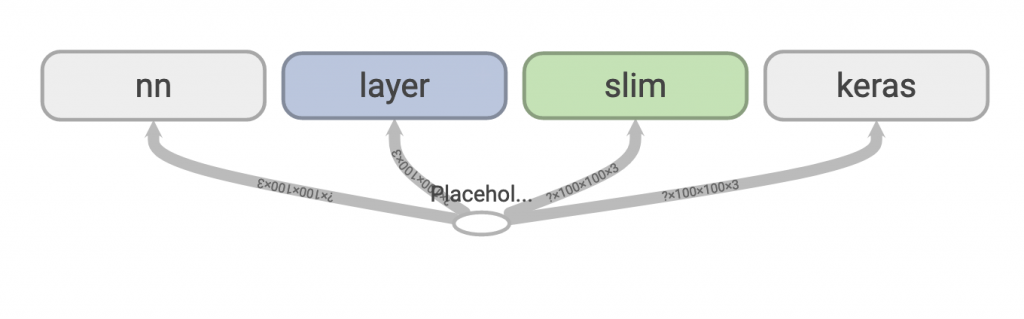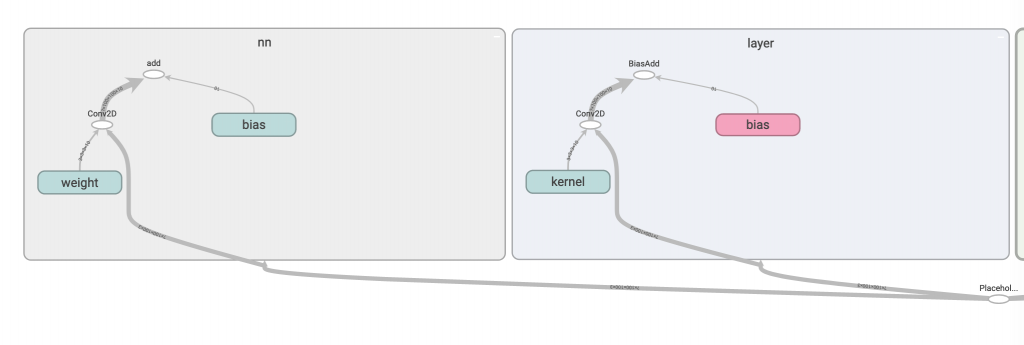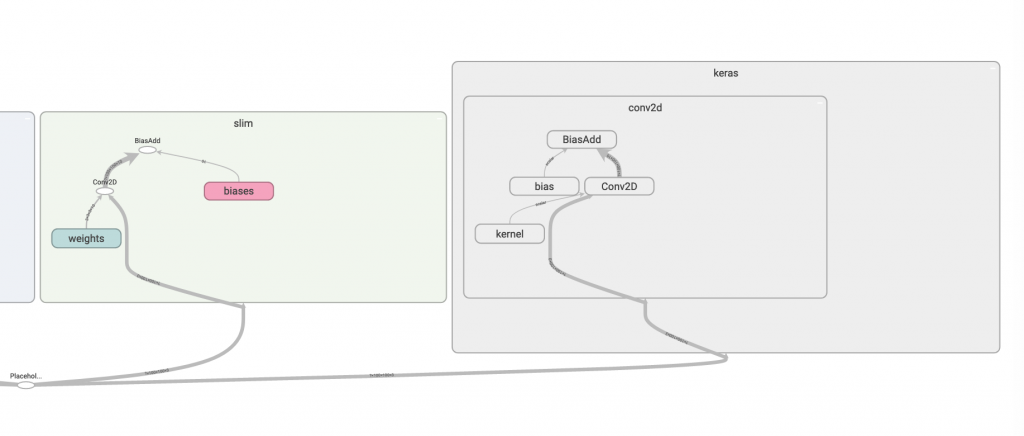#DAY 5
0

## 【05】tensorflow 的 convolution 方式好多種，我該用哪個...

``````if __name__ == '__main__':
node = tf.placeholder(shape=[None, 100, 100, 3], dtype=tf.float32)

nn_out = nn(node)  # 第一種方式
layer_out = layer(node)  # 第二種方式
slim_out = slim(node)  # 第三種方式
keras_out = keras(node)  # 第四種方式

tf.summary.FileWriter(OUTPUT_PATH, graph=tf.get_default_graph())

image = np.expand_dims(image, 0)

with tf.Session() as sess:
sess.run(tf.global_variables_initializer())
sess.run(tf.local_variables_initializer())

nn_result, layer_result, slim_result, keras_result = \
sess.run([nn_out, layer_out, slim_out, keras_out], feed_dict={node: image})

print(f'nn shape: {nn_result.shape}')  # 第一種方式shape
print(f'layer shape: {layer_result.shape}')  # 第二種方式shape
print(f'slim shape: {slim_result.shape}')  # 第三種方式shape
print(f'keras shape: {keras_result.shape}')  # 第四種方式shape
``````

``````def nn(input_node):
with tf.variable_scope('nn'):
w = tf.get_variable(
name='weight',
shape=[FILTER_SIZE, FILTER_SIZE, 3, NUM_FILTERS], dtype=tf.float32)
b = tf.get_variable(
name='bias',
shape=[NUM_FILTERS],
dtype=tf.float32)
out = tf.nn.conv2d(input_node, filter=w, strides=(1, 1), padding='SAME')
out = out + b

return out
``````

tf.nn 其實是我最常使用的實作方式，但是他非常的底層，所以很多人因此排斥它，你要自己宣告 variable，然後用 tf.nn.conv2d 合併。雖然它最麻煩，但是有時你想高度客製化時，就會需要它，而且其實用久就會習慣了ＸＤ

``````def layer(input_node):
out = tf.layers.conv2d(
input_node,
NUM_FILTERS,
FILTER_SIZE,
strides=STRIDES,
name='layer')

return out
``````

``````def slim(input_node):
out = tf.contrib.slim.conv2d(
input_node,
NUM_FILTERS,
FILTER_SIZE,
stride=STRIDES,
activation_fn=None,
scope='slim')

return out
``````

slim 是 google 出的一個高階API，但是我自己其實沒什麼用過 slim，所以這邊就簡單寫個範例給大家當參考，但要注意的是，目前 slim 似乎漸漸被淡化，新版的 tensorflow 2.0 建議你使用 keras 這高階的API來實作。

``````def keras(input_node):
model = tf.keras.Sequential([
tf.keras.layers.Conv2D(
NUM_FILTERS, FILTER_SIZE, strides=STRIDES, padding='same')
], name='keras')

return model(input_node)
``````

Tensorflow 2.0 的新星，但我好像底層有點寫久了，用起來不太習慣，所以這邊我用了 tf.keras.Sequential 來把這單獨一層 conv 包裝起來，其實我也不確定這樣的寫法好不好，單純想 demo 這樣包起來後的 graph 會長怎樣。tf.nn 和 layers 細部圖:slim 和 keras 細部圖:github原始碼